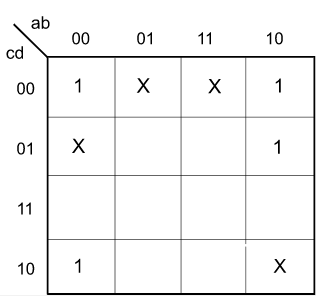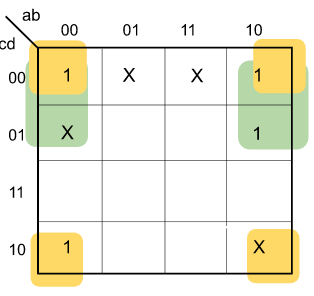# Logic functions and Minimization

 Question 1
Which one of the following expressions does NOT represent exclusive NOR of x and y?xy+x'y'x⊕y'x'⊕yx'⊕y'

Question 1-Explanation:
By Definition of XNOR,So Option-A is correct. Also by Definition of XOR,Option-B isSo Option-B is also correct. Option-C isOption-C is also correct. Option-D x'⊕y' = x''y' + x'y'' = xy' + x'y = x⊕y ≠ x⊙y Therefore option (D) is false. This explanation is provided by Chirag Manwani.
 Question 2

What is the minimal form of the Karnaugh map shown below? Assume that X denotes a don’t care term.b'd'b'd' + b'c'b'd' + a'b'c'd'b'd' + b'c' + c'd'

Question 2-Explanation:

There are two prime implicants in the following K-Map-Prime Implicant highlighted in Green =Prime Implicant highlighted in Orange =So the Boolean expression is-Therefore option (B) is correct.

 Question 3
Which one of the following circuits is NOT equivalent to a 2-input XNOR (exclusive NOR) gate?ABCD

Question 3-Explanation:
All options except D produce XOR as described below :Question 4
The simplified SOP (Sum Of Product) form of the boolean expression (P + Q' + R') . (P + Q' + R) . (P + Q + R') is(P'.Q + R')(P + Q'.R')(P'.Q + R)(P.Q + R)

Question 4-Explanation:
See following : (P+Q'+R').(P+Q'+R).(P+Q+R') =From the K-map, POS form is : P + Q'.R'
 Question 5
The minterm expansion of f(P, Q, R) = PQ + QR' + PR' ism2 + m4 + m6 + m7m0 + m1 + m3 + m5m0 + m1 + m6 + m7m2 + m3 + m4 + m5

Question 5-Explanation:
K-map,= PQ + QR’ + PR’
= PQ(R+R’) + (P+P’)QR’ + P(Q+Q’)R’
= PQR + PQR’ +PQR’ +P’QR’ + PQR’ + PQ’R’
= PQR(m7) + PQR'(m6)+P’QR'(m2) +PQ’R'(m4)
= m2 + m4 + m6 + m7 
Option (A) is correct.
 Question 6
What is the minimum number of gates required to implement the Boolean function (AB+C)if we have to use only 2-input NOR gates?2345

Question 6-Explanation:
AB+C = (A+C)(B+C) = ((A+C)' + (B+C)')' So, '3' 2-input NOR gates are required.
 Question 7
In the Karnaugh map shown below, X denotes a don't care term. What is the minimal form of the function represented by the Karnaugh map?A)B)C)D)ABCD

Question 7-Explanation:One group consists of (0000, 0010, 1000, 1010) which gives b’d’ Other group is (0000, 0001, 1000, 1001) which gives a’d’ So, solution is b’d’ + a’d’
 Question 8
Given f1, f3 and f in canonical sum of products form (in decimal) for the circuitA)m(4, 6)

B)m(4, 8)

C)m(6, 8)

D)m(4, 6, 8)ABCD

Question 8-Explanation:
From logic diagram  we have f=f1.f2+f3 f=m(4,5,6,7,8).f2+m(1,6,15)----(1) from eq(1) we need to find such f2 so that we can get  f=m(1,6,8,15) eq(1)  says we can get m(1,6,15) from f3 ,so only  8 left now from option (a,b,d) we get (4,6), (4,8) ,(4,6,8) respectively for m(4,5,6,7,8)f2 which is not required as m4 is undesired. But option (C) m(4,5,6,7,8)(6,8)+(1,6,15)
• m(6,8)+m(1,6,15)
• m(1,6,8,15)an
Option (C) is correct.
 Question 9
If P, Q, R are Boolean variables, then (P + Q')(PQ' + PR)(P'R' + Q') simplifiesPQ'PR'PQ' + RPR'' + Q

Question 9-Explanation:
Step by step explanation :
= (P + Q’)(PQ’ + PR)(P’R’ + Q’)
= (PPQ’ + PPR + PQ’Q’ + PQ’R) (P’R’ + Q’)
= (PQ’ + PR + PQ’ + PQ’R) (P’R’ + Q’)
= (PP’Q’R’ + PP’R’R + PP’Q’R’ + PP’Q’RR’ + PQ’Q’ + PQ’R + PQ’Q’ + PQ’Q’R)
= (0 + 0 + 0 + 0 + PQ’ + PQ’R + PQ’ + PQ’R)
= PQ’ + PQ’R
= PQ'(1 + R)
= PQ’ 
So, option (A) is correct.
 Question 10
Consider the following Boolean function of four variables: f(w,x,y,z) = ∑(1,3,4,6,9,11,12,14) The function is:independent of one variables.independent of two variables.independent of three variables.dependent on all the variables.

Question 10-Explanation:On solving K-MAP we get ZX’+XZ’ so  it is independent of w,y Ans (B) part.
There are 104 questions to complete.
• Last Updated : 19 Nov, 2018